Boyle's law applies to processes that keep the gas mass and temperature constant, on the other hand, Charles' law keeps the gas mass and pressure constant. Let us now consider a process in which pressure, volume and temperature change.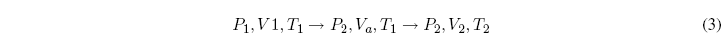We are going to divide the process into two stages, in the first the temperature is kept constant and in the second the pressure.
Let's apply Boyle's law to the first stage: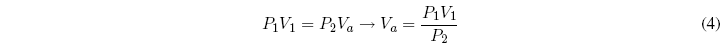Now we will apply Charles's law to the second stage:Equating both equations: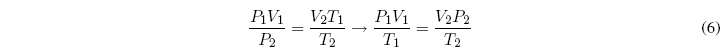A m = constant, where m is the mass of the gas, it can be written: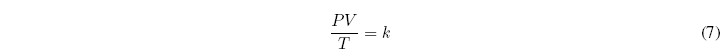Let us now consider the variation of the mass, keeping the pressure and temperature of the gas constant, we arrive at the relationship:, since the mass is directly related to the number of moles, the previous equation can be written as: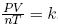, where n represents the number of moles of the gas.

The constant of proportionality k, is a universal constant, called the ideal gas constant and it is customary to represent it by R. Thus, the equation of state of ideal gases can be written as:Where R = 0.082 atml/Kmol = 8.314 J/molK = 1.987 cal/molK
This law is only exact in the limit of zero pressure.